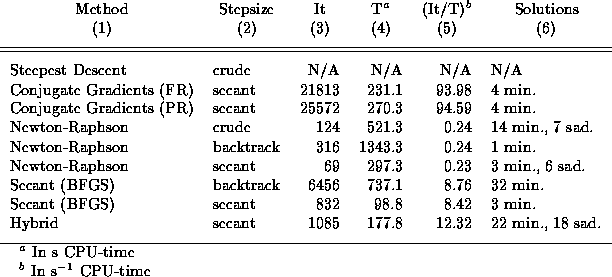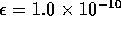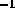Next: A Physical Discussion Up: Orbits of Galaxies in Previous: The System Parameters

## Extended Evaluation of Optimizing Methods

The evaluation of the optimizing methods is executed in the same manner as in the Section 5.3; i.e. with 100 different initial values of the coefficients equally spaced between 0 and 10. The results are given in Table 5.5, which is equivalent to Table 5.3, with the exception of the exclusion of the implementations of some of the optimizing methods declared not suitable in Section 5.3. Therefore, neither the Steepest Descent method using the linear search nor the Secant method using the DFP updating formula will be tested in this section.

The method of Steepest Descent showed in Section 5.3 signs of being a very poor optimizing method, at least when applied to the AVP. As expected from theory (see Subsection 4.3.1), the convergence of the method slows down when closing in on the solution, a behavior that is most noticeable when dealing with badly scaled systems. The system considered here is very badly scaled, even when using the Bernoulli-shaped trial functions. It has a condition number as large as, which results in the Steepest Descent not converging after as much as 70 hours CPU-time. Hence the ``N/A'' in Table 5.5.Table: Comparison of the remaining optimizing methods that past the test in Section 5.3 for a system of 22 galaxies, with 660 variables, and termination set at. The columns are: (1) method, (2) stepsize determiner, (3) average value of the number of iterations for 100 different runs, (4) CPU-time in seconds used for the average value in column (3), (5) iterations per seconds in CPU-time, and (6) types of solutions located by the method.

The Conjugate Gradients method, on the other hand, seems to be a rather efficient method. It spends a large number of iterations before locating the solution to a reasonable accuracy, but each iteration is very fast, so that the total CPU-time used is acceptable. The method has been proven to be superior to the Steepest Descent, as is the consensus from numerical literature. When it comes to the choice of updating formula, the tendency shown in Section 5.3 is indicated here as well: the Fletcher-Reeves formula seems to hold a hand over the Polak-Ribière formula. Nevertheless, it may very well be that the situation changes when applied to different optimizing problems. Both implementations managed to locate four different minimum points.

The Newton-Raphson method showed signs of being a very efficient method, at least for the rather small system considered in Section 5.3, but it was also mentioned that this could change for larger system. So is the case: The calculation and inversion of the now rather large Hessian matrix is indeed elaborate and time consuming.The number of iterations is still modest compared to the other optimizing methods, but the total computation time is substantially lengthened. This is especially the case with the implementation using the backtracking scheme, which, with the exception of the Steepest Descent method, has the longest average computation time of all the optimizing methods in consideration. The implementation that spent the least amount of computing time was the the one with the secant stepsize algorithm. This is somewhat surprising, since the linear searches are usually thought of as being too elaborate to give any gain in efficiency. The reason why the secant method doesn't affect the efficiency notably can almost be read directly from column (5) in Table 5.5. The number of iterations per second is similar for all the three different implementations, which means that the speed of each iteration is almost independent of the choice of stepsize determiner. The majority of time is taken up by the computation and the inversion of the Hessian matrix, and the efficiency is therefore seriously enhanced by the fast convergence caused by linear searches.

The three different implementations of the Newton-Raphson method does not only show diversity in computing time--also the number of distinct solutions located is notably different. The implementation using the secant and the crude stepsize determiner finds 9 and 21 allowed stationary points, respectively, while the backtrack implementation only ends up at one. The only similarity is that all three implementations end up at 100 different solutions starting from the 100 different initial values, implying a large number of bad solutions: 91 with the secant stepsize determiner, 79 using the crude method, and as much as 99 using backtracking. All in all, based on both computing time and number of different allowed solutions, the implementation using backtracking seems to be a very poor choice for the type of system treated here. The question which of the other two versions of the Newton-Raphson method to chose, is a bit more open. The implementation using the secant method is preferable when it comes to efficiency, but is not able to locate as many stationary points as the the crude stepsize implementation.

The Secant method proved itself once again to be a very efficient method, especially when using the secant stepsize determiner, which was the fastest of all the methods tested. On average, it spent only 1.6 minutes CPU time in locating a stationary point. A problem with this version is that it is only able to find three minimum points, while the version using backtracking located as many as 32. The problem with the latter is that the computation time on average is as large as 12.3 minutes.

The last method tested, the Hybrid method, spend on average a marginally longer time in converging than the Secant method using the secant stepsize determiner, but has the advantage of finding a considerably larger number of allowed stationary values, 40 all in all. Similarly to the Newton-Raphson method, almost every initial value gave a distinct solution, resulting in 56 bad solutions.

The results based on the testing in this section are as follows. The optimizing methods which one safely can discard are: The method of Steepest Descent, The Conjugate Gradients method using the Polak-Ribière updating formula, and Newton-Raphson using the backtracking scheme. This leaves us with the Conjugate Gradients method implementation using the Fletcher-Reeves formula, Newton-Raphson using the crude and the secant stepsize determiners, the Secant method using the backtracking and the linear search, and finally, the Hybrid method. For both the Newton-Raphson and the Secant method, one of the two different remaining implementations have the advantage over the other either when it comes to speed or to the number of solutions detected. By looking at all the methods with their different implementations as a whole, it should be possible to settle on a method that either is the fastest or is able to locate the largest number of acceptable solutions. Preferably we would like to have a method with both these qualities, but as seen in this section, none of the optimizing methods considered here has that ability. The method that found its way to the solution in the least amount of time was the Secant method using linear searches, but at the same time it found the smallest number of solutions. The second fastest was the Hybrid method, which also happen to be the one able to locate the largest number of different permissible solutions, both minimum and saddle points. The Hybrid method seems therefore altogether to be the best choice of optimizing method. But, if one is only interested in a large amount of minimum points, the Conjugate Gradients method using the Polak-Ribière formula or the Secant method using linear searches are preferable. The choice of method is all in all a choice of convenience, but with the Hybrid method as the recommended method for general use.

It should be noted that in addition to the large diversity in the number of solutions located by the different implementations of the optimizing methods, a considerable number of solutions found by one method were not detected by the other, and vica versa. For example, of the 22 minimum points located by the Hybrid method, none were included in the set of 32 minimum points detected by the Secant method using backtracking. This should indicate that there are at least 54 minimum points for this system, and that none of the optimizing methods were able to locate them all starting from the 100 different initial values used here.

I close this subsection by mentioning that there are ways to limit the mulitivalued behavior of the system, and thereby also reducing the number of bad solutions. One way is simply to chose a system of mass tracers that is sparser; i.e. galaxies that are close to each other are treated as a group, thereby limiting the possibility of orbit-crossing. Onwards, one can make sure that solutions that allow two galaxies coming closer than a certain distance are rejected. P-96 set this limit to 30 kpc in proper units, assuming that a pair that passes closer than that would likely have merged. P-96 also proposed that one could discard solutions that do not give a good enough fit of predicted to the observed velocities for the adjacent dwarf galaxies. Peebles accepted the solutions that gave a fit better than 75 km s.Next: A Physical Discussion Up: Orbits of Galaxies in Previous: The System Parameters

Trond Hjorteland
Mon Jul 5 02:59:28 MET DST 1999